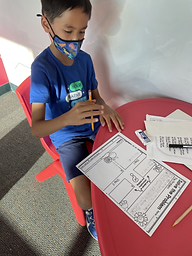## Ms. Caitlyn

### Target 1​

###### Lesson Type:

New

Measurment

:

Time

Calculate elapsed time.

###### 1:

Understand that time is the duration of an event from beginning to end.

###### 2:

Understand that elapsed time can be found by find the total amount of time that passes between the starting and ending time of an event.

4th

###### Vocabulary:

Time, Elapsed, Minute, Hour

Activities:

Students used number lines to determine how much time has a elapsed from two given times.

Students completed a Movie Night activity to calculate how much time has elapsed from beginning to end of the movie.

Students worked on page 19 of their workbook.### Home Exploration

###### Guiding Questions:## Absent Students:

### Target 2

:

###### 1:

Identify the correct arithmetic processes based on the information presented in word problems.

###### 2:

Find the key words in word problems that indicate the correct arithmetic process to use: Addition: add, altogether, both, combined, in all, plus, sum, total; Subtraction: difference, fewer, how many/much more, left, less, minus, remains; Multiplication: times, product, each, twice; and Division: how many in each, divided by, quotient, goes into, split evenly.

###### 3:

Find and use the needed information in a word problem to solve.

5th

###### Vocabulary:

Word Problem, Addition, Subtraction, Multiplication, Division

Activities:

Students began by doing a brain dump of all the words they knew related to addition, subtraction, multiplication, and addition.

Students identified key components in word problems to solve them using the CUBES method: (C)ircle the numbers, (U)nderline the question, (B)ox key words, (E)liminate what you don't need, (S)olve the problem.### Home Exploration

###### Guiding Questions:### Target 3

:

###### Vocabulary:

Activities:### Home Exploration